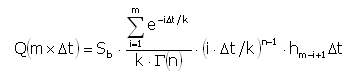﻿ Output > High flood mapping

# HIGH FLOOD MAPPING

Apart from the climax flow it may be useful to know the flow' s evolution, in the section referred to, depending on the period.

The construction of such a graphic is obtained with the method proposed by Nesh:where:

Q(m·Δt)        = the instantaneous flow Δt, with m variable from 1 to N;

N                = the maximum number of intervals considered;

Δt                   = the temporary time interval (1 hour);

m             = interval number;

Γ(n)       = the gamma function;

Sb           = basin's area [Km²];

hm-i+1   = the effective inflow [mm] in the interval (m-i+1);

k, n         = basin's characteristic coefficients.

For estimating the coefficients k and n there are available several correlations, the program uses:

Nash (1960)
n and k are known by determining the m1 and m2 parameters with:with:

m1 = 27.6·A0.3·ib-0.3

m2 = 0.41·L-0.1

where:

A = basin's area expressed in square miles;

L = water course length, prolongated, in mules;

ib = basin's medium inclination in parts on 10000.

Mc Sparran (1968)

Mc Sparran calculates:with:

tp = 5.52·A0.208·i-0.447

k1 = 3.34·A0.297·i-0.354

where:

A= basin's area expressed in square miles;

i = the medium inclination of the water course in parts on miles.